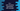# C++ setw function explanation with example## C++ setw function explanation with example:

setw is defined in header. setw function is used to set the field width for any output operation. In this post, we will learn how to use setw in C++ with an example.

## Definition of setw:

setw is defined as like below:

``setw(int n)``

Where, n is the width, or number of characters used as field width.

### Return value of setw:

setw doesn’t return anything. It can be used only to manipulate the stream.

## Example of setw:

Let’s try setw with different examples.

### Example 1: setw with a higher width:

Let’s take a look at the below example:

``````#include <iostream>
#include <iomanip>
using namespace std;

int main()
{
cout<<setw(8);
cout<<"hello"<<endl;
}``````

Here,

• We need to import iomanip since we are using setw.
• we have assigned 8 as the width using setw. The next line is printing the hello word, which has 5 characters.
• So, it will add three blank spaces to the left of this word.
``   hello``

### Example 2: setw with a smaller width:

Let’s try setw with another example. In this example, we are passing a smaller value to setw:

``````#include <iostream>
#include <iomanip>
using namespace std;

int main()
{
cout<<setw(0);
cout<<"hello"<<endl;
}``````

It will not change anything. Since, length of hello is greater than 0, it will print hello without adding any spacing to left.

``hello``

### Right aligning using setw:

setw is useful if we want to print values aligning to the right.

For example, if we have an array of strings, we can find the string with the largest length and use setw for that length. Next, if we print the values, all will be right aligned.

``````#include <iostream>
#include <iomanip>
using namespace std;

int main()
{
string days[] = {"Sun", "Mon", "Tues", "Wed", "Thurs", "Fri", "Sat"};

int largestLength = 0;

for (int i = 0; i < 7; i++)
{
if (days[i].length() > largestLength)
{
largestLength = days[i].length();
}
}

for (int i = 0; i < 7; i++)
{
cout << setw(largestLength);
cout << days[i] << endl;
}
}``````

Here,

• days is an array of strings.
• largestLength is an int variable to hold the length of the largest element of the array days.
• We are iterating through the elements of the days one by one and updating the largest length variable. Once this loop will end, largestLength will hold the length of the word with maximum number of characters.
• The last for loop is used to print the values of days. It prints each value and before printing, it uses setw to assign the width to the largest length.

If you run the above program, it will print the below output:

``````  Sun
Mon
Tues
Wed
Thurs
Fri
Sat``````

As you can see, all are right-aligned.